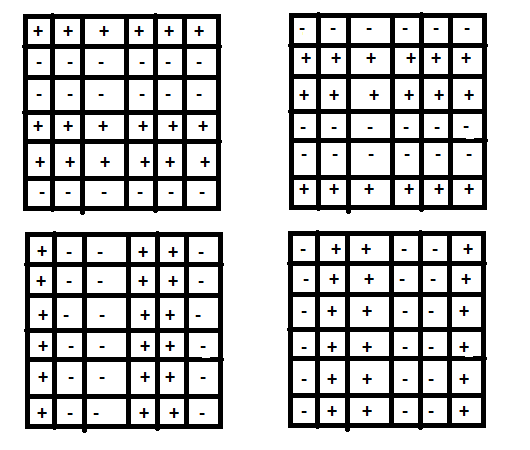Related Articles

# Puzzle | The neighbour

• Difficulty Level : Hard
• Last Updated : 18 Jan, 2018

Sahil has got a challenge problem from his friend Akhil. Sahil has to find all values of n for which one can fill an n × n table with +’s and -’s (one per cell) so that every cell has exactly one neighbor with the opposite sign. Two cells are considered neighbors if they are either in the same row or the same column. Can you help out Sahil to find such values of n ?

Solution :
The puzzle has a solution if n is even, and it does not have a solution if n is odd. If n is even, one can fill the top row with pluses, rows 2 and 3 with minuses, rows 4 and 5 with pluses again, and so on, until the last row is filled with pluses. Under this scheme, every cell will have exactly one neighbor with the opposite sign either above or below the cell in question. Of course, as alternative solutions, one can interchange the pluses and minuses in the previous solution or have every column, rather than every row, contain the same sign. As an example if we take a 6 x 6 table, then the possible solutions can be :EXPLANATION :
1). If a plus is put in the upper left corner, we have to put a plus and a minus in the neighboring cells, as every cell will have exactly one neighbor with the opposite sign.
2). Consider the case when a plus is put in its row neighbor and a minus in its column neighbor; the other case is symmetric.
3). Thus, let us prove that if pluses are put in the first two cells of row 1 and a minus in the first cell of row 2, we will have to fill the remaining cells of row 1 with pluses and the remaining cells of row 2 with minuses.
4). Since the first cell in the second row already has a neighbor with a plus above it, the second cell in row 2 will have to contain a minus. But then the third cell in row 1 will have to contain a plus since the second cell in row 1 already has a neighbor with a minus below it.
5). By the same argument, all the remaining cells of row 1 will have to contain a plus, and all the remaining cells in row 2 will have to contain a minus.
6). But then all the cells in row 3 must contain minuses because their neighbors in row 2 already have the plus neighbors in row 1.
7). This implies that row 3 cannot be last but must be followed by row 4 filled with all pluses. Row 4 can be either last or must be followed with the all plus row, and so on.

This proves that when n is even ,the puzzle has no other solutions than those described above. And it does not have a solution when n is odd.

My Personal Notes arrow_drop_up